# 算法原理¶

DeepLabV3+主要有两个创新点。

## 编解码器¶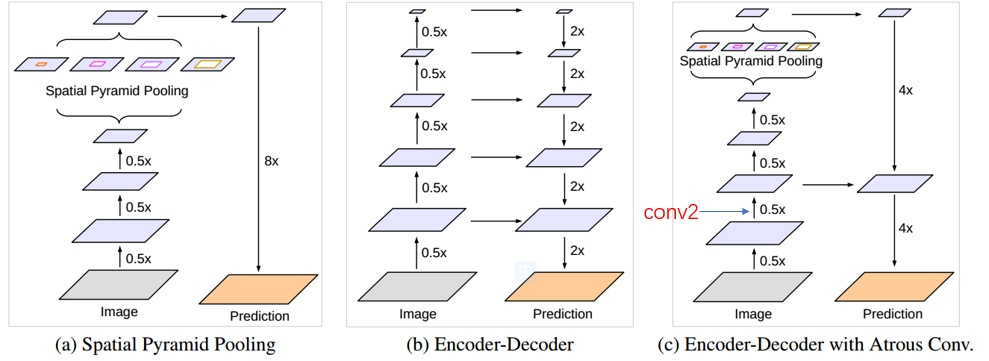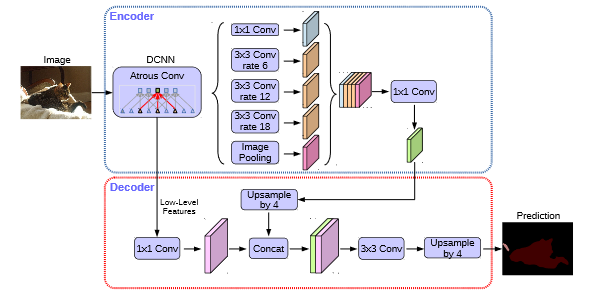## 更改主干网络¶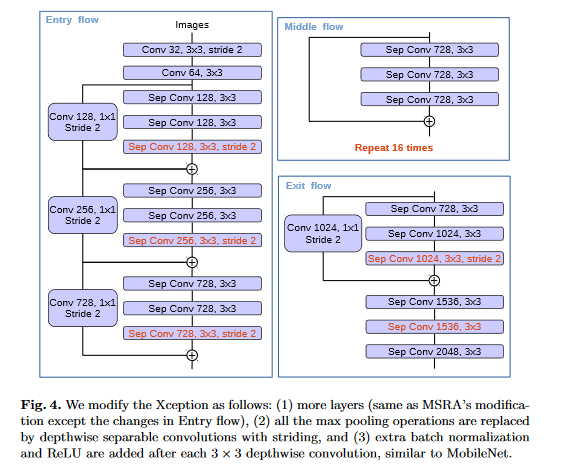• 更深的Xception结构，不同的地方在于不修改entry flow network的结构，为了快速计算和有效的使用内存
• 所有的max pooling结构被stride=2的深度可分离卷积代替
• 每个3x3的depthwise convolution都跟BN和Relu

# 实验¶

## 使用1*1卷积少来自低级feature的通道数¶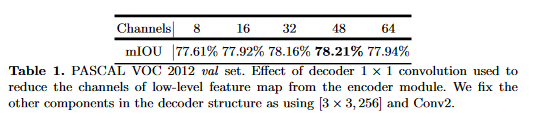## 使用3*3卷积逐步获取分割结果¶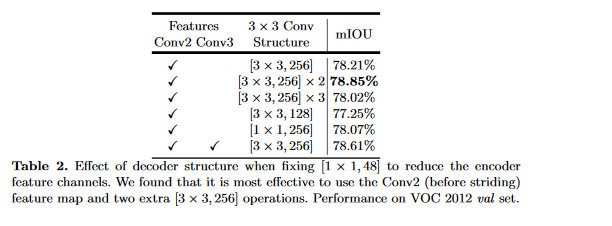# Backbone为ResNet101¶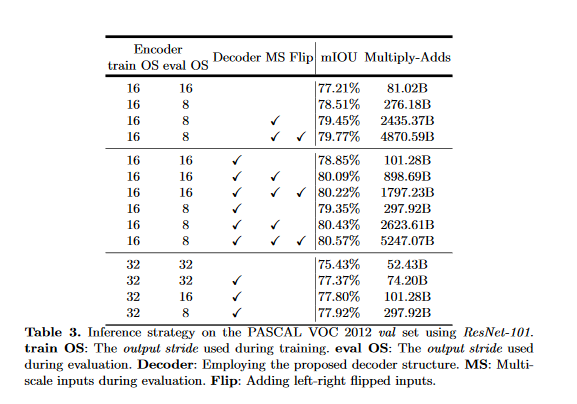# Backbone为Xception¶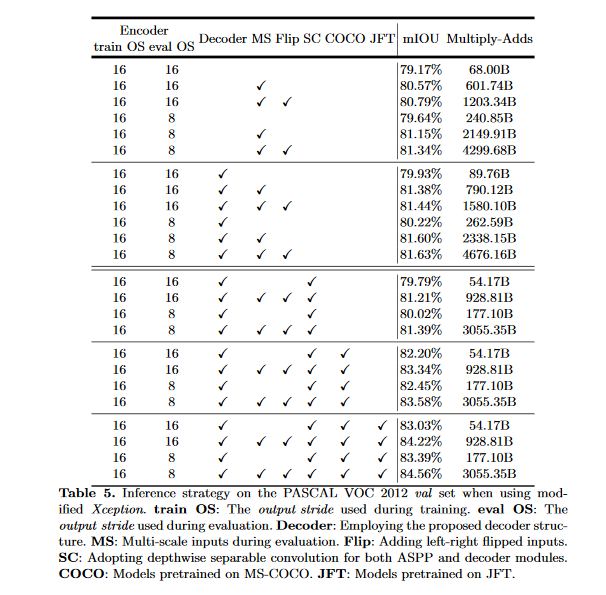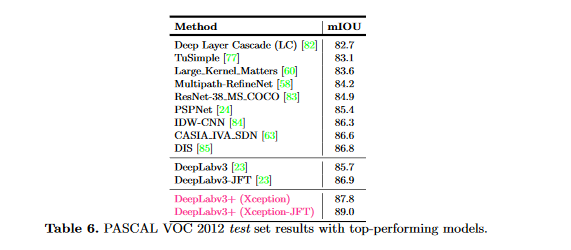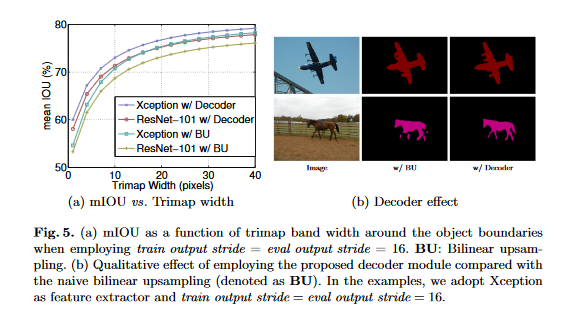# 代码实现¶

from __future__ import absolute_import, print_function

from collections import OrderedDict

import torch
import torch.nn as nn
import torch.nn.functional as F

from .deeplabv3 import _ASPP
from .resnet import _ConvBnReLU, _ResLayer, _Stem

class DeepLabV3Plus(nn.Module):
"""
DeepLab v3+: Dilated ResNet with multi-grid + improved ASPP + decoder
"""

def __init__(self, n_classes, n_blocks, atrous_rates, multi_grids, output_stride):
super(DeepLabV3Plus, self).__init__()

# Stride and dilation
if output_stride == 8:
s = [1, 2, 1, 1]
d = [1, 1, 2, 4]
elif output_stride == 16:
s = [1, 2, 2, 1]
d = [1, 1, 1, 2]

# Encoder
ch = [64 * 2 ** p for p in range(6)]
self.layer1 = _Stem(ch)
self.layer2 = _ResLayer(n_blocks, ch, ch, s, d)
self.layer3 = _ResLayer(n_blocks, ch, ch, s, d)
self.layer4 = _ResLayer(n_blocks, ch, ch, s, d)
self.layer5 = _ResLayer(n_blocks, ch, ch, s, d, multi_grids)
self.aspp = _ASPP(ch, 256, atrous_rates)
concat_ch = 256 * (len(atrous_rates) + 2)
self.add_module("fc1", _ConvBnReLU(concat_ch, 256, 1, 1, 0, 1))

# Decoder
self.reduce = _ConvBnReLU(256, 48, 1, 1, 0, 1)
self.fc2 = nn.Sequential(
OrderedDict(
[
("conv1", _ConvBnReLU(304, 256, 3, 1, 1, 1)),
("conv2", _ConvBnReLU(256, 256, 3, 1, 1, 1)),
("conv3", nn.Conv2d(256, n_classes, kernel_size=1)),
]
)
)

def forward(self, x):
h = self.layer1(x)
h = self.layer2(h)
h_ = self.reduce(h)
h = self.layer3(h)
h = self.layer4(h)
h = self.layer5(h)
h = self.aspp(h)
h = self.fc1(h)
h = F.interpolate(h, size=h_.shape[2:], mode="bilinear", align_corners=False)
h = torch.cat((h, h_), dim=1)
h = self.fc2(h)
h = F.interpolate(h, size=x.shape[2:], mode="bilinear", align_corners=False)
return h

if __name__ == "__main__":
model = DeepLabV3Plus(
n_classes=21,
n_blocks=[3, 4, 23, 3],
atrous_rates=[6, 12, 18],
multi_grids=[1, 2, 4],
output_stride=16,
)
model.eval()
image = torch.randn(1, 3, 513, 513)

print(model)
print("input:", image.shape)
print("output:", model(image).shape)


# 参考文章¶

https://blog.csdn.net/u011974639/article/details/79518175